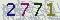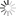﻿ Explicit Numerical Approximations for Stochastic Differential Equations in Finite and Infinite Horizons-山东大学新闻网### Explicit Numerical Approximations for Stochastic Differential Equations in Finite and Infinite Horizons

Explicit Numerical Approximations for Stochastic Differential Equations in Finite and Infinite Horizons

2021年9月29日 19:00

Solving stochastic differential equations (SDEs) numerically, explicit Euler-Maruyama (EM) schemes are used most frequently under global Lipschitz conditions for both drift and diffusion coefficients. In contrast, without imposing the global Lipschitz conditions, implicit schemes are often used for SDEs but require additional computational effort; along another line, tamed EM schemes and truncated EM schemes have been developed recently. Taking advantages of being explicit and easily implementable, truncated EM schemes are proposed in this paper. Convergence of the numerical algorithms is studied, and pth moment boundedness is obtained. Furthermore, asymptotic properties of the numerical solutions such as the exponential stability in pth moment and stability in distribution are examined. Several examples are given to illustrate our findings.

【作者：杨媛    来自：数学与交叉科学研究中心    编辑：新闻网工作室    责任编辑：王涵 蒋晓涵 　】

 验证码看不清楚,换张图片 共0条评论    共1页   当前第1页 拖动光标可翻页查看更多评论

### 最新发布### 新闻排行• 山大日记
• 山大人物
• 视点微信
• 互动话题
• 视点图志
• 精彩视频